GATE  >  GATE Numerical Computation Aptitude (Quant) Questions - 2

# GATE Numerical Computation Aptitude (Quant) Questions - 2

Test Description

## 10 Questions MCQ Test General Aptitude for GATE | GATE Numerical Computation Aptitude (Quant) Questions - 2

GATE Numerical Computation Aptitude (Quant) Questions - 2 for GATE 2023 is part of General Aptitude for GATE preparation. The GATE Numerical Computation Aptitude (Quant) Questions - 2 questions and answers have been prepared according to the GATE exam syllabus.The GATE Numerical Computation Aptitude (Quant) Questions - 2 MCQs are made for GATE 2023 Exam. Find important definitions, questions, notes, meanings, examples, exercises, MCQs and online tests for GATE Numerical Computation Aptitude (Quant) Questions - 2 below.
Solutions of GATE Numerical Computation Aptitude (Quant) Questions - 2 questions in English are available as part of our General Aptitude for GATE for GATE & GATE Numerical Computation Aptitude (Quant) Questions - 2 solutions in Hindi for General Aptitude for GATE course. Download more important topics, notes, lectures and mock test series for GATE Exam by signing up for free. Attempt GATE Numerical Computation Aptitude (Quant) Questions - 2 | 10 questions in 15 minutes | Mock test for GATE preparation | Free important questions MCQ to study General Aptitude for GATE for GATE Exam | Download free PDF with solutions
 1 Crore+ students have signed up on EduRev. Have you?
GATE Numerical Computation Aptitude (Quant) Questions - 2 - Question 1

### A train that is 280 metres long, travelling at a uniform speed, crosses a platform in 60 seconds and passes a man standing on the platform in 20 seconds. What is the length of the platform in metres?

Detailed Solution for GATE Numerical Computation Aptitude (Quant) Questions - 2 - Question 1

The train with length 280m passes by a man standing on platform in 20s.
The speed of train is V = 280/20 = 14m/s
Let the length of platform be L
(L + 280 )/60 = 14
L + 280 = 60×14
L = 840 - 280
L = 560 metres

GATE Numerical Computation Aptitude (Quant) Questions - 2 - Question 2

### The sum of eight consecutive odd numbers is 656. The average of four consecutive even numbers is 87. What is the sum of the smallest odd number and second largest even number?

Detailed Solution for GATE Numerical Computation Aptitude (Quant) Questions - 2 - Question 2

The sum of 8 consecutive odd numbers is 656
⇒ Average of the 8 odd numbers = 656/8 = 82
the odd numbers immediately preceding and succeeding 82 are 81 are 83 respectively and these will be the middle numbers.
Therefore, we see that 4th and 5th odd numbers are 81 and 83 respectively.
Thus we can easily obtain the odd numbers as 75, 77, 79, 81, 83, 85, 87, 89 and smallest odd number as 75
the average of four consecutive even numbers is 87
As seen earlier, 86 and 88 will be 2nd and 3rd even numbers.
i.e., 84, 86, 88 and 90 will be the even numbers and the second largest even number is 88
Therefore required sum is 75 + 88 = 163

GATE Numerical Computation Aptitude (Quant) Questions - 2 - Question 3

### A man can row at 8 km per hour in still water. If it takes him thrice as long to row upstream, as to row downstream, then find the stream velocity in km per hour.

Detailed Solution for GATE Numerical Computation Aptitude (Quant) Questions - 2 - Question 3

Speed of man (m) = 8km/h
Let the speed of stream be s
According to the question:
Speed of man upstream = S1= m − s
Speed of man downstream = S= m + s
Speed = Distance/Time
Here, since the distance D is same
D = S× T1
D = S× T2
S× T= S× T2
S1/S= T2/T= 3
m + s = 3(m − s)
or, 8 + s = 3(8 − s)
⇒ s = 4km/h

GATE Numerical Computation Aptitude (Quant) Questions - 2 - Question 4

A batch of one hundred bulbs is inspected by testing four randomly chosen bulbs. The batch is rejected if even one of the bulbs is defective. A batch typically has five defective bulbs. The probability that the current batch is accepted is _________

Detailed Solution for GATE Numerical Computation Aptitude (Quant) Questions - 2 - Question 4

Probability for one bulb to be non defective is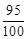∴ Probabilities that none of the bulbs is defectives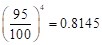Binomial Problem with n = 4 and p(not defective) = 0.95
P(x = 4 non defective) = 0.95 x 4 = 0.8145

GATE Numerical Computation Aptitude (Quant) Questions - 2 - Question 5

A car travels 8 km in the first quarter of an hour, 6 km in the second quarter and 16 km in the third quarter. The average speed of the car in km per hour over the entire journey is

Detailed Solution for GATE Numerical Computation Aptitude (Quant) Questions - 2 - Question 5

This is a simple formula based one.
Average speed = total distance/ total time
Total distance = 8+6+16 = 30 km
Total time taken = 3 quarters of an hour = ¾ hr
Average speed = total distance/ total time = 30/(3/4) = 40 kmph

GATE Numerical Computation Aptitude (Quant) Questions - 2 - Question 6

You are given three coins: one has heads on both faces, the second has tails on both faces, and the third has a head on one face and a tail on the other. You choose a coin at random and toss it, and it comes up heads. The probability that the other face is tails is

Detailed Solution for GATE Numerical Computation Aptitude (Quant) Questions - 2 - Question 6

This problem is an application of bayes' theorem.
This theorem shold be applied when the outcome is already determined .
so in the problem we need to calculate all possible ways a head could show up.
We have HH TH TT
probability of head showing up on the first coin is chance of first coin getting picked x chance of head showing up = 1/3 x 1 = 1/3
Simlarly for the 2nd coin the probablility is 1/3  x 1/2
For the 3rd since it doesnt have a head we dont really care
Now to calculate the prob that the head came from the 2 nd coin applying bayes theorem
p(H) = 1/3(1/2) / 1/3 + 1/3(1/2) = 1/3

GATE Numerical Computation Aptitude (Quant) Questions - 2 - Question 7

It takes 30 minutes to empty a half-full tank by draining it at a constant rate. It is decided to simultaneously pump water into the half-full tank while draining it. What is the rate at which water has to be pumped in so that it gets fully filled in 10 minutes?

Detailed Solution for GATE Numerical Computation Aptitude (Quant) Questions - 2 - Question 7

Lets say capacity of tank is 1 litre
draining rate = 0.5litre/30minutes = 1/60 litre/min
let filling rate = x litre/min
in 1 min tank gets x - (1/60) litre filled.
to fill the remaining half part we need 10mins
x - 1/60 litre → 1min
0.5 litre → 10 mins
0.5/(x - 1/60) = 10
solving, we get x = 4/60
which is 4 times more than draining rate.

GATE Numerical Computation Aptitude (Quant) Questions - 2 - Question 8

A firm producing air purifiers sold 200 units in 2012. The following pie chart presents the share of raw material, labour, energy, plant & machinery, and transportation costs in the total manufacturing cost of the firm in 2012. The expenditure on labour in 2012 is Rs. 4,50,000. In 2013, the raw material expenses increased by 30% and all other expenses increased by 20%. If the company registered a profit of Rs. 10 lakhs in 2012, at what price (in Rs.) was each air purifier sold?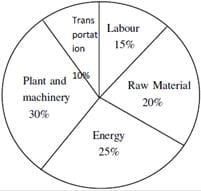Detailed Solution for GATE Numerical Computation Aptitude (Quant) Questions - 2 - Question 8

Given that expenditure on labour in 2012 is Rs, 4,50,000 which is 15% of cost of total manufacturing cost of firm

Total Manufacturing Cost x 0.15 = 4,50,000

Total Mfg. Cost = 4,50,000 / 0.15

= 30,00, 000

Total Profit is = 10,00,000

Total Sale obtained from 200 purifier  = manufaturing cost + profit = 40,00,000

So cost of one purifier = 40,00,000 / 200

= Rs. 20,000

GATE Numerical Computation Aptitude (Quant) Questions - 2 - Question 9

In the summer of 2012, in New Delhi, the mean temperature of Monday to Wednesday was 41 °C and of Tuesday to Thursday was 43 °C. If the temperature on Thursday was 15% higher than that of Monday, then the temperature in °C on Thursday was

Detailed Solution for GATE Numerical Computation Aptitude (Quant) Questions - 2 - Question 9

Let the temperature on Monday, Tuesday, Wednesday and Thursday be respectively as TM, TTU, TW, TTH,
So, from the given data we have
The mean temperature of Monday to Wednesday was 41oC => (M+T+W)/3 = 41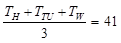...(1)
The mean temperature of Tuesday to Thursday was 43oC=> (T+W+Th)/3 = 43
and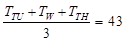...(2)
Also, as the temperature on Thursday was 15% higher than that of Monday
i.e.,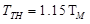…(3)
After Solving The above equations (1), (2) and (3) we obtain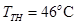Hence correct option is C.

GATE Numerical Computation Aptitude (Quant) Questions - 2 - Question 10

Find the sum to n terms of the series 10 + 84 + 734 + _____.

Detailed Solution for GATE Numerical Computation Aptitude (Quant) Questions - 2 - Question 10

Check from the options by substituting n = 1, 2…
For n = 1, all options are right
For n = 2, sum must be 10 + 84 = 94.
Only option D is correct for this

Given Series in AGP (Arithematic Geomatric Progression)

10 + 84 + 734 + ... = (91 +1) + (92 + 3) + (93 + 5)+...

For solving AGP progression we need to find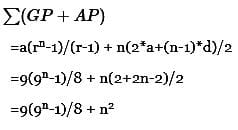## General Aptitude for GATE

73 videos|86 docs|108 tests
 Use Code STAYHOME200 and get INR 200 additional OFF Use Coupon Code
Information about GATE Numerical Computation Aptitude (Quant) Questions - 2 Page
In this test you can find the Exam questions for GATE Numerical Computation Aptitude (Quant) Questions - 2 solved & explained in the simplest way possible. Besides giving Questions and answers for GATE Numerical Computation Aptitude (Quant) Questions - 2, EduRev gives you an ample number of Online tests for practice

## General Aptitude for GATE

73 videos|86 docs|108 tests